# 辉光（Glare,Glow）

辉光效果，也就是Glare，Glow效果。它的主要作用是模拟一些场景中发光物体周边的朦胧模糊的发光效果，有点类似于PS中的外发光。先来看一看这种效果的实际图片，给大家一个感性的认识。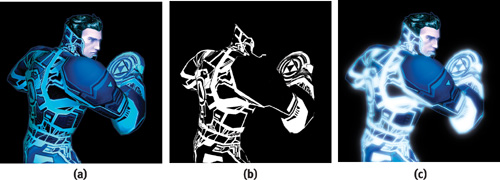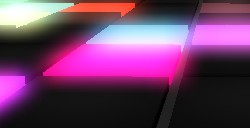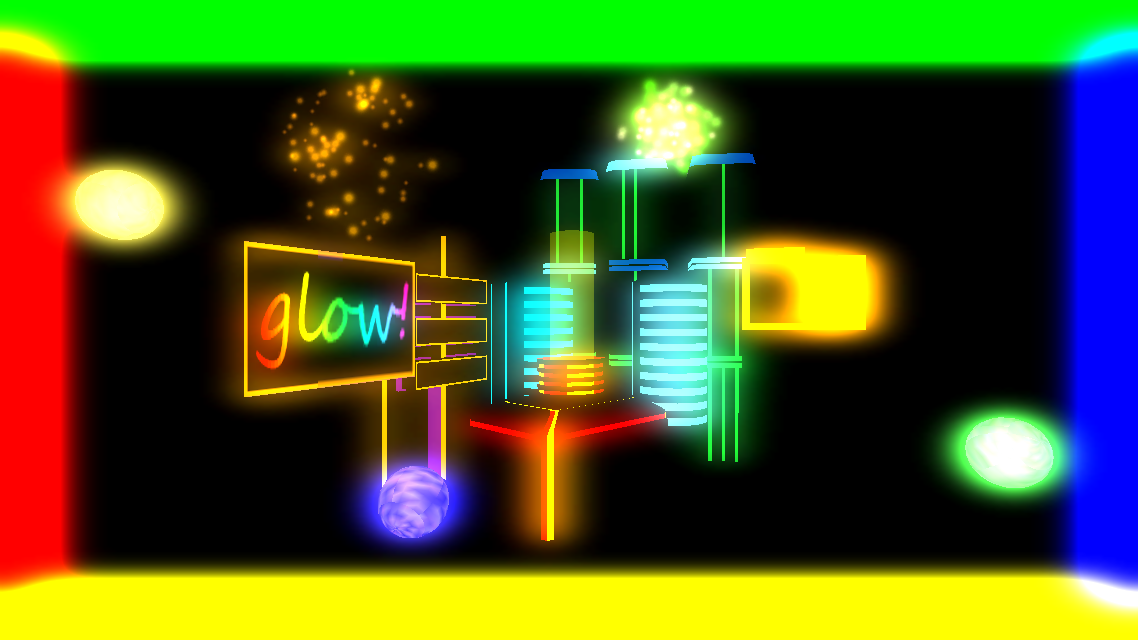上面图片给大家展示了辉光效果能够带来的视觉体验。为场景中的发光物体添加辉光效果，能够实现灯光的朦胧感觉。当然，为了整体画面的和谐也不能乱用此效果，否则就给人一种很俗气的感觉。

# 原理

下面给大家讲述下这种效果的实现方式。其实说白了它的实现原理很简单，我们只要对要添加辉光效果的物体进行一次模糊操作，然后将模糊过后的图片与原先的图片进行Alpha Blend，就能够得到上面所示的效果。操作如图所展示的那样（这里为了简便，只在场景中绘制Glare物体，并且只添加了些许光照，没有添加其他效果）：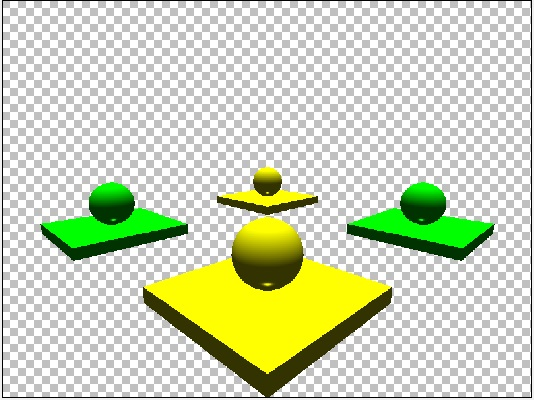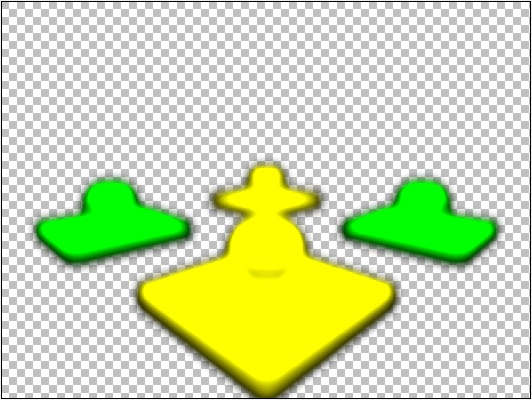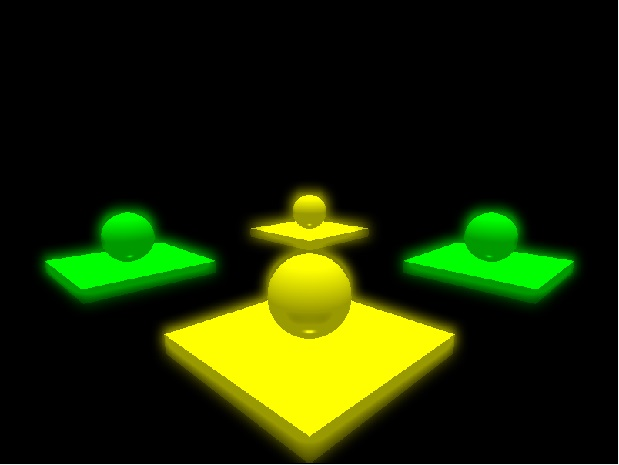上面三张图片展示了绘制一个简单辉光效果的整体步骤。原理是不是十分的简单？？？大家可以自己在PS等图像处理软件中，直接模拟看看是否能够得出这种效果来。

# OpenGL实现

在讲述具体的实现之前，我们需要先了解一些基本的知识点。在了解了这些知识点的基础之上，我们才能够实现这种效果。

## 渲染到纹理（Render to Texture, RTT）

在图形学处理中，我们经常需要对渲染好的场景进行一些处理。也就是说，我们需要一种方式，能够让我们通过图形API（OpenGL, DirectX）绘制的场景保存到我们指定的内存（显存）中去，以便于我们后期对他进行一些处理。而在OpenGL中，我们能够很容易的实现这一点。这种技术在

## 后处理(Post-processing)

后处理在图形学中经常被用到。我们在游戏中绘制的场景，往往是没有办法仅仅依靠光照模型计算就能够实现出来的。很多的特殊效果，都是通过对渲染好的场景进行图像空间的后期出理来得到。比如本篇文章中实现的辉光效果，就使用了这种技术。还有很多其他在图像空间进行后处理的特效，感兴趣的同学可以阅读Real-time Rendering中关于此技术的章节。

## 图像模糊(Blur)

在前面讲述辉光效果整体绘制流程的时候，讲到了我们需要对原场景图像进行模糊操作。那么怎么样才能够实现对图像的模糊操作了？

在图像处理中，我们经常使用的模糊是基于

高斯模糊在图像处理的时候，是使用2维的高斯分布函数对一个像素的上下左右包围的像素进行采样来实现的。我们假设模糊的半径为20个像素，按照高斯模糊的原始计算方式，每一个像素都需要计算(2 * 20) ^ 2次采样。这在图像处理上面可以接受，但是对于实时性的游戏来说，计算量就有点大了。

为此，人们想出了一种优化的方法来实现高斯模糊。对于一个像素，它的模糊半径为20，那么我们可以分两次进行模糊，一次是横向的模糊计算，一次是纵向的模糊计算。也就是将模糊操作从O(N^2)降低到了2 * O(N)。下图展示了如何进行这样的操作：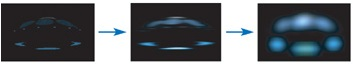从图中，我们可以看到，我们先对原场景图进行一次横向的模糊计算，此次计算我们只需要采用一维的高斯分布进行计算即可。然后对横向模糊之后的图像进行纵向模糊，与横向模糊一样，使用一维的高斯分布函数进行。这样就能够得到一张高斯模糊之后的图像，它的模糊效果不比二维高斯模糊效果来的差。

## OpenGL绘制流程

下面就以我的代码中的绘制流程来向大家展示如何在OpenGL中绘制一个辉光效果：

[cpp]
view plain

1. void glb_display() {
2.     glb_draw_normal_scene();
3.
4.     glb_draw_render_target_gauss_blur_h();
5.
6.     glb_draw_render_target_gauss_blur_v();
7.
8.     glb_draw_blend();
9.
10.     glutSwapBuffers();
11. }

上面的代码给出了我绘制的流程：

(1).绘制正常场景 -- 此处绘制的场景保存到一张纹理A中，并且复制A纹理到B纹理中

(4)经过前三步之后，A纹理保存了模糊之后的图像，B纹理保存了原图像，然后画一个矩形，把这两张贴图都传递进入，进行Alpha混合计算。

[cpp]
view plain

1. light_pixel.vs
[cpp]
view plain

1. //--------------------------------------------------------------------
2. // Declaration: Copyright (c), by i_dovelemon, 2016. All right reserved.
3. // Author: i_dovelemon[1322600812@qq.com]
4. // Date: 2016 / 06 / 29
5. // Brief: Phong lights's vertex shader
6. //--------------------------------------------------------------------
7. #version 330
8.
9. in vec3 vertex;
10. in vec3 normal;
11.
12. uniform mat4 proj;
13. uniform mat4 view;
14. uniform mat4 world;
15. uniform mat4 trans_inv_world;
16.
17. out vec3 vs_vertex;
18. out vec3 vs_normal;
19.
20. void main() {
21.     gl_Position = proj * view * world * vec4(vertex, 1.0);
22.     vs_vertex = (world * vec4(vertex, 1.0)).xyz;
23.     vs_normal = (trans_inv_world * vec4(normal, 0.0)).xyz;
24. }

[cpp]
view plain

1. light_pixel.ps
[cpp]
view plain

1. //--------------------------------------------------------------------
2. // Declaration: Copyright (c), by i_dovelemon, 2016. All right reserved.
3. // Author: i_dovelemon[1322600812@qq.com]
4. // Date: 2016 / 06 / 29
5. // Brief: Phong lights's pixel shader
6. //-------------------------------------------------------------------
7. #version 330
8.
9. in vec3 vs_vertex;
10. in vec3 vs_normal;
11.
12. out vec4 color;
13.
14. uniform vec3 parallel_light_dir;
15. uniform vec3 parallel_light_ambient;
16. uniform vec3 parallel_light_diffuse;
17. uniform vec3 parallel_light_specular;
18.
19. uniform vec3 material_ambient;
20. uniform vec3 material_diffuse;
21. uniform vec3 material_specular;
22. uniform float material_specular_pow;
23.
24. uniform vec3 eye_pos;
25.
26. vec3 calc_diffuse(vec3 light_vec, vec3 normal, vec3 diffuse, vec3 light_color) {
27.     float ratio = dot(light_vec, normal);
28.     ratio = max(ratio, 0.0);
29.     return diffuse * light_color * ratio;
30. }
31.
32. vec3 calc_specular(vec3 light_vec, vec3 normal, vec3 view_vec, vec3 specular, vec3 light_color, float pow_value) {
33.     vec3 ref_light_vec = reflect(light_vec, normal);
34.     float ratio = dot(ref_light_vec, view_vec);
35.     ratio = max(ratio, 0.0);
36.     ratio = pow(ratio, pow_value);
37.
38.     return specular * light_color * ratio;
39. }
40.
41. void main() {
42.     vec3 light_vec = -parallel_light_dir;
43.     vec3 normal = normalize(vs_normal);
44.     vec3 view_vec = normalize(eye_pos - vs_vertex);
45.
46.     vec3 ambient = material_ambient * parallel_light_ambient;
47.     vec3 diffuse = calc_diffuse(light_vec, normal, material_diffuse, parallel_light_diffuse);
48.     vec3 specular = calc_specular(light_vec, normal, view_vec, material_specular, parallel_light_specular, material_specular_pow);
49.
50.     color = vec4(ambient + diffuse + specular, 1.0);
51. }

[cpp]
view plain

1. gauss_blur.vs
[cpp]
view plain

1. //--------------------------------------------------------------------
2. // Declaration: Copyright (c), by i_dovelemon, 2016. All right reserved.
3. // Author: i_dovelemon[1322600812@qq.com]
4. // Date: 2016 / 06 / 29
5. // Brief: Gauss blur pass through vertex shader
6. //--------------------------------------------------------------------
7. #version 330
8.
9. in vec3 vertex;
10. in vec2 texcoord;
11.
12. out vec2 vs_texcoord;
13.
14. void main() {
15.     gl_Position = vec4(vertex, 1.0);
16.     vs_texcoord = texcoord;
17. }

[cpp]
view plain

1. gauss_blurh.ps
[cpp]
view plain

1. //--------------------------------------------------------------------
2. // Declaration: Copyright (c), by i_dovelemon, 2016. All right reserved.
3. // Author: i_dovelemon[1322600812@qq.com]
4. // Date: 2016 / 06 / 29
5. // Brief: Gauss blur horizontal pass shader
6. //--------------------------------------------------------------------
7. #version 330
8.
9. in vec2 vs_texcoord;
10. out vec4 color;
11.
12. uniform sampler2D tex;
13. uniform float tex_width;
14.
15. uniform float gauss_num;
16.
17. void main() {
18.     color = texture2D(tex, vs_texcoord) * gauss_num;
19.     float step = 1.0 / tex_width;
20.
21.     for (int i = 1; i < 21; i++) {
22.         if (vs_texcoord.x - i * step >= 0.0) {
23.             color += texture2D(tex, vec2(vs_texcoord.x - i * step, vs_texcoord.y)) * gauss_num[i];
24.         }
25.
26.         if (vs_texcoord.x + i * step <= 1.0) {
27.             color += texture2D(tex, vec2(vs_texcoord.x + i * step, vs_texcoord.y)) * gauss_num[i];
28.         }
29.     }
30. }

[cpp]
view plain

1. gauss_blurv.ps
[cpp]
view plain

1. //--------------------------------------------------------------------
2. // Declaration: Copyright (c), by i_dovelemon, 2016. All right reserved.
3. // Author: i_dovelemon[1322600812@qq.com]
4. // Date: 2016 / 06 / 29
5. // Brief: Gauss blur vertical pass shader
6. //--------------------------------------------------------------------
7. #version 330
8.
9. in vec2 vs_texcoord;
10. out vec4 color;
11.
12. uniform sampler2D tex;
13. uniform float tex_height;
14.
15. uniform float gauss_num;
16.
17. void main() {
18.     color = texture2D(tex, vs_texcoord) * gauss_num;
19.     float step = 1.0 / tex_height;
20.
21.     for (int i = 0; i <21; i++) {
22.         if (vs_texcoord.y - i * step >= 0.0) {
23.             color += texture2D(tex, vec2(vs_texcoord.x, vs_texcoord.y - i * step)) * gauss_num[i];
24.         }
25.
26.         if (vs_texcoord.y + i * step <= 1.0) {
27.             color += texture2D(tex, vec2(vs_texcoord.x, vs_texcoord.y + i * step)) * gauss_num[i];
28.         }
29.     }
30. }

[cpp]
view plain

1. float glb_gauss_num(int x) {
2.     float pi = 3.1415927f;
3.     float e = 2.71828f;
4.     float theta = 0.1f;
5.     float theta2 = theta * theta;
6.     float temp1 = 1.0f / (theta * sqrt(2 * pi));
7.     float temp2 = pow(e, -(x * x) / 2 * theta2);
8.     return temp1 * temp2;
9. }
10.
11. void glb_calc_gauss_nums() {
12.     g_GaussNum = 1.0f;
13.
14.     for (int32_t i = 1; i < sizeof(g_GaussNum) / sizeof(g_GaussNum); i++) {
15.         g_GaussNum[i] = glb_gauss_num(i);
16.     }
17.
18.     float total = 0.0f;
19.     for (int32_t i = 0; i < sizeof(g_GaussNum) / sizeof(g_GaussNum); i++) {
20.         total += g_GaussNum[i];
21.     }
22.
23.     for (int32_t i = 0; i < sizeof(g_GaussNum) / sizeof(g_GaussNum); i++) {
24.         g_GaussNum[i] = g_GaussNum[i] / total;
25.     }
26. }

[cpp]
view plain

1. blend.vs
[cpp]
view plain

1. //--------------------------------------------------------------------
2. // Declaration: Copyright (c), by i_dovelemon, 2016. All right reserved.
3. // Author: i_dovelemon[1322600812@qq.com]
4. // Date: 2016 / 06 / 29
5. // Brief: Blend pass through vertex shader
6. //--------------------------------------------------------------------
7. #version 330
8.
9. in vec3 vertex;
10. in vec2 texcoord;
11.
12. out vec2 vs_texcoord;
13.
14. void main() {
15.     gl_Position = vec4(vertex, 1.0);
16.     vs_texcoord = texcoord;
17. }

[cpp]
view plain

1. blend.ps
[cpp]
view plain

1. //--------------------------------------------------------------------
2. // Declaration: Copyright (c), by i_dovelemon, 2016. All right reserved.
3. // Author: i_dovelemon[1322600812@qq.com]
4. // Date: 2016 / 06 / 29
5. // Brief: Alpha blend shader
6. //--------------------------------------------------------------------
7. #version 330
8.
9. in vec2 vs_texcoord;
10. out vec4 color;
11.
12. uniform sampler2D blur_tex;
13. uniform sampler2D scene_tex;
14.
15. void main() {
16.     vec4 blur_color = texture2D(blur_tex, vs_texcoord);
17.     vec4 scene_color = texture2D(scene_tex, vs_texcoord);
18.     color = blur_color * 0.5 + scene_color * 0.5;
19. }

最后的混合计算，我选取了模糊贴图的一半加上场景贴图的一半来进行混合操作。最终的效果如下图所示：# 总结

所以，本片文章仅仅是为了向大家讲述辉光算法的基本原理，至于如何整合到你们自己的项目中去，需要大家自己来思考！希望本篇文章能够帮助到你们！

# 参考文献

http://http.developer.nvidia.com/GPUGems/gpugems_ch21.html GPU Gems - Chapter 21.Real-time Glow